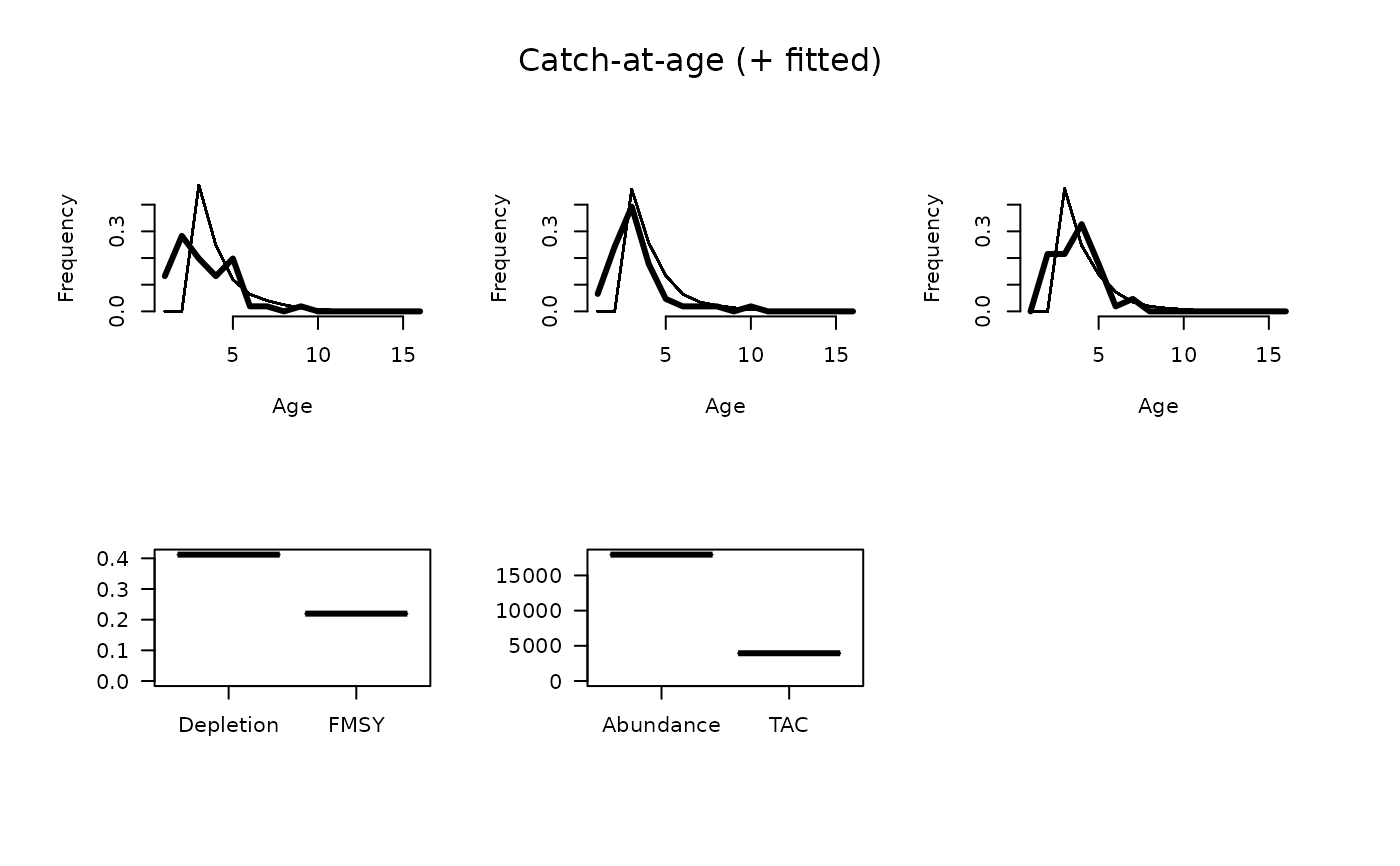A stock reduction analysis (SRA) model is fitted to the age-composition from the last 3 years (or less if fewer data are available)

CompSRA(x, Data, reps = 100, plot = FALSE)

CompSRA4010(x, Data, reps = 100, plot = FALSE)

## Arguments

x

A position in the data object

Data

A data object

reps

The number of stochastic samples of the MP recommendation(s)

plot

Logical. Show the plot?

## Value

An object of class Rec-class with the TAC slot populated with a numeric vector of length reps

## Details

A stock reduction analysis (SRA) model is fitted to the age-composition from the last 3 years (or less if fewer data are available) assuming a constant total mortality rate (Z) and used to estimate current stock depletion (D), $$F_\textrm{MSY}$$, and stock abundance (A).

Abundance is estimated in the SRA. $$F_{\textrm{MSY}}$$ is calculated assuming knife-edge vulnerability at the age of full selection.

The TAC is calculated as $$F_\textrm{MSY} A$$. CompSRA4010 uses a 40-10 harvest control rule to reduce TAC at low biomass.

## Functions

• CompSRA: TAC is FMSY x Abundance

• CompSRA4010: With a 40-10 control rule based on estimated depletion

## Required Data

See Data-class for information on the Data object

CompSRA: CAA, Cat, L50, LFC, LFS, MaxAge, Mort, steep, vbK, vbLinf, vbt0, wla, wlb

CompSRA4010: CAA, Cat, L50, LFC, LFS, MaxAge, Mort, steep, vbK, vbLinf, vbt0, wla, wlb

## Rendered Equations

See Online Documentation for correctly rendered equations

T. Carruthers

## Examples

if (FALSE) {
CompSRA(1, MSEtool::SimulatedData, plot=TRUE)
}

CompSRA4010(1, MSEtool::SimulatedData, plot=TRUE)
#> TAC (median)
#>     3944.184